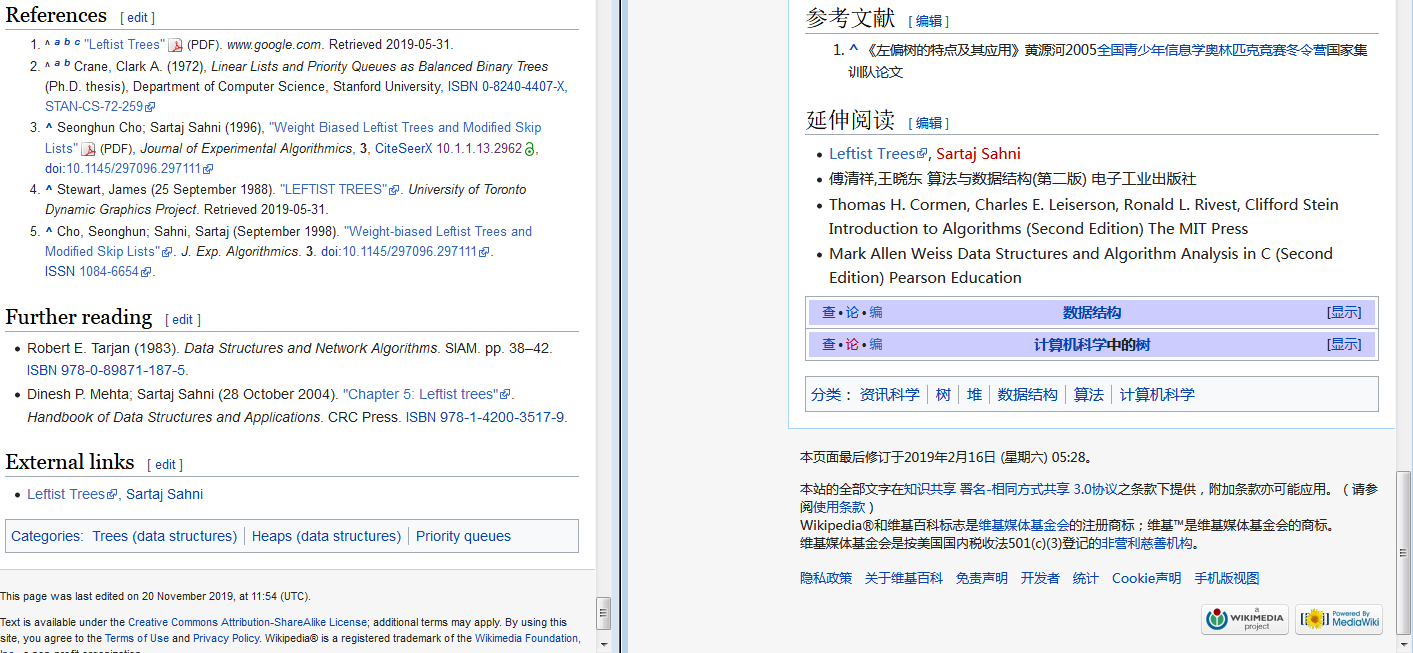# <总结>左偏树

（啊那个中文版太扯了）

1. 我们假设原始左偏树的根分别为A和B，其中v_A \ge v_B（例子为小根堆）。否则，我们可以交换A和B，使上述条件成立；
2. 将B与A的右子树合并；
3. 因为上一次操作可能会改变A的右子树的s值。为了维护左偏树属性，在每次B与A的右子树合并并且A的右子树被修改完成后，我们还应检查右子树的s值是否大于左子树的s值，如果右子树的s值大于左子树的s值，我们就交换左右子树。
4. 如果这个节点只有一个子节点，那么这个子节点应该是左儿子
Node *merge(Node *x, Node *y)
{
if (x == 0) return y;
if (y == 0) return x;
if (x->v > y->v || (x->v == y->v && x > y)) std::swap(x, y);
//啊，或右边的表达式是洛谷板子的要求：当堆里有多个最小值时，优先删除原序列的靠前的
x->r = merge(x->r, y);
x->r->f = x;//方便找root
if ((x->l ? x->l->d : -1) < x->r->d) std::swap(x->l, x->r);
x->d = (x->r) ? (x->r->d + 1) : 1;
return x;
}


Node *get(Node *p)
{
if (p->f) p = p->f;
return p;
}


Node *get(Node *p)
{
if (p->f != p) return p->f = get(p->f);
return p;
}

//main()
for (int i = 1; i <= n; ++i)
scanf("%d", &nd[i].v), nd[i].f = nd + i;//fa[i] = i

//main():merge
Node *a = get(nd + x), *b = get(nd + y);
if (a==b)
continue;
a->f = b->f = merge(a, b);

//main():pop
ro = find(nd + x);
ro->v = 0;
ro->f = merge(ro->l, ro->r);//这里！
if (ro->l)
ro->l->f = ro->f;
if (ro->r)
ro->r->f = ro->f;


（已知堆顶）最值查询 O(1)